# 用户增长实战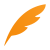## 三.商业模式的区别

### 3.2 海盗指标

“海盗指标”这个术语由风险投资人戴夫·麦克卢尔创造，得名于五个成功创业关键元素的首字母缩写。将公司最需要关注的指标分为五大类：获取用户（Acquisition）、提高活跃度（Activation）、提高留存率（Retention）、获取营收（Revenue）和自传播（Referral），简称AARRR。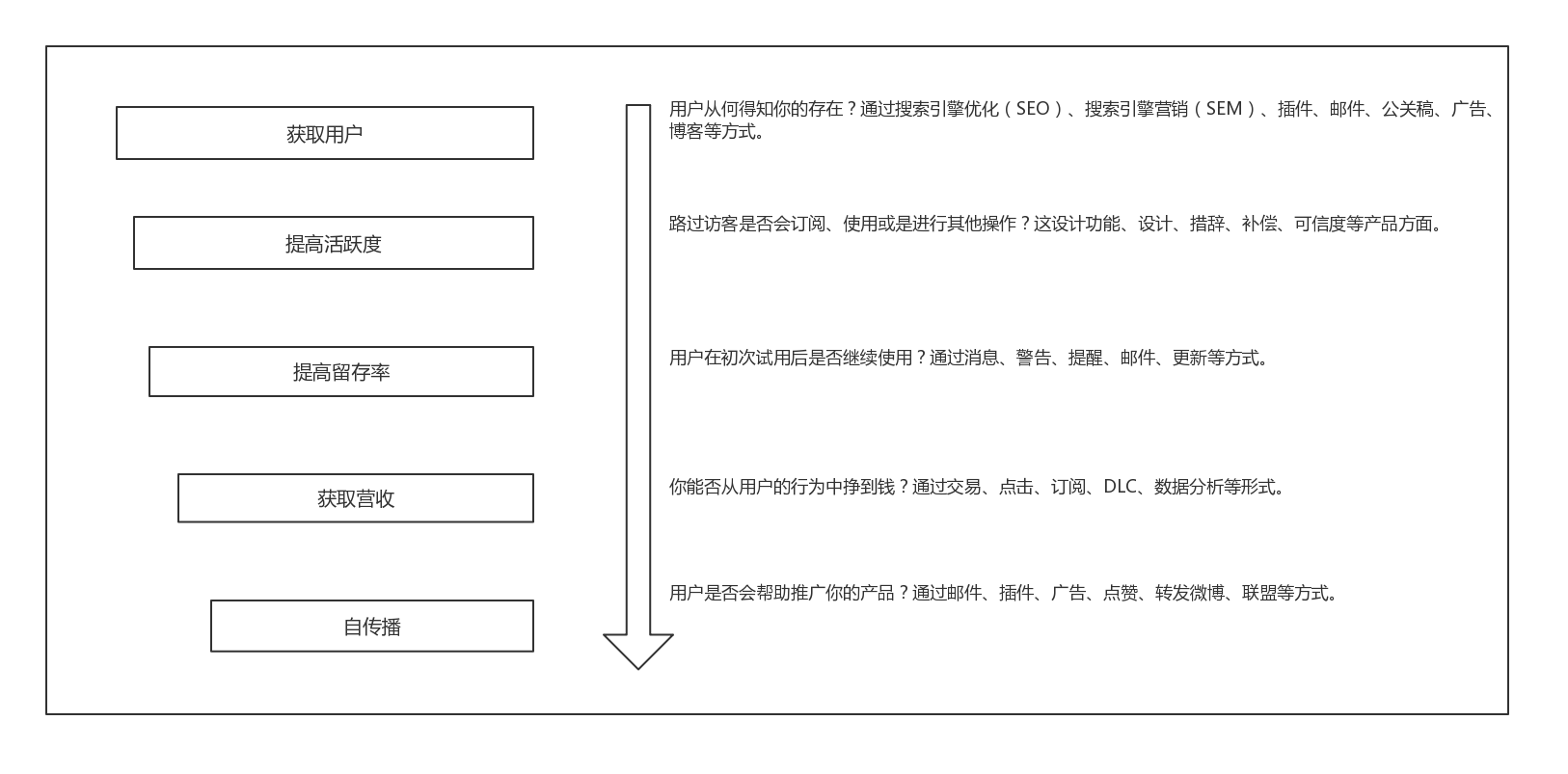### 3.3 增长引擎

《精益数据分析》中提到 3 种增长引擎：黏着式增长引擎、病毒式增长引擎、付费式增长引擎。埃里克 · 莱斯提出了驱动创业增长的三大引擎，它们都有各自对应的关键绩效指标（KPI）。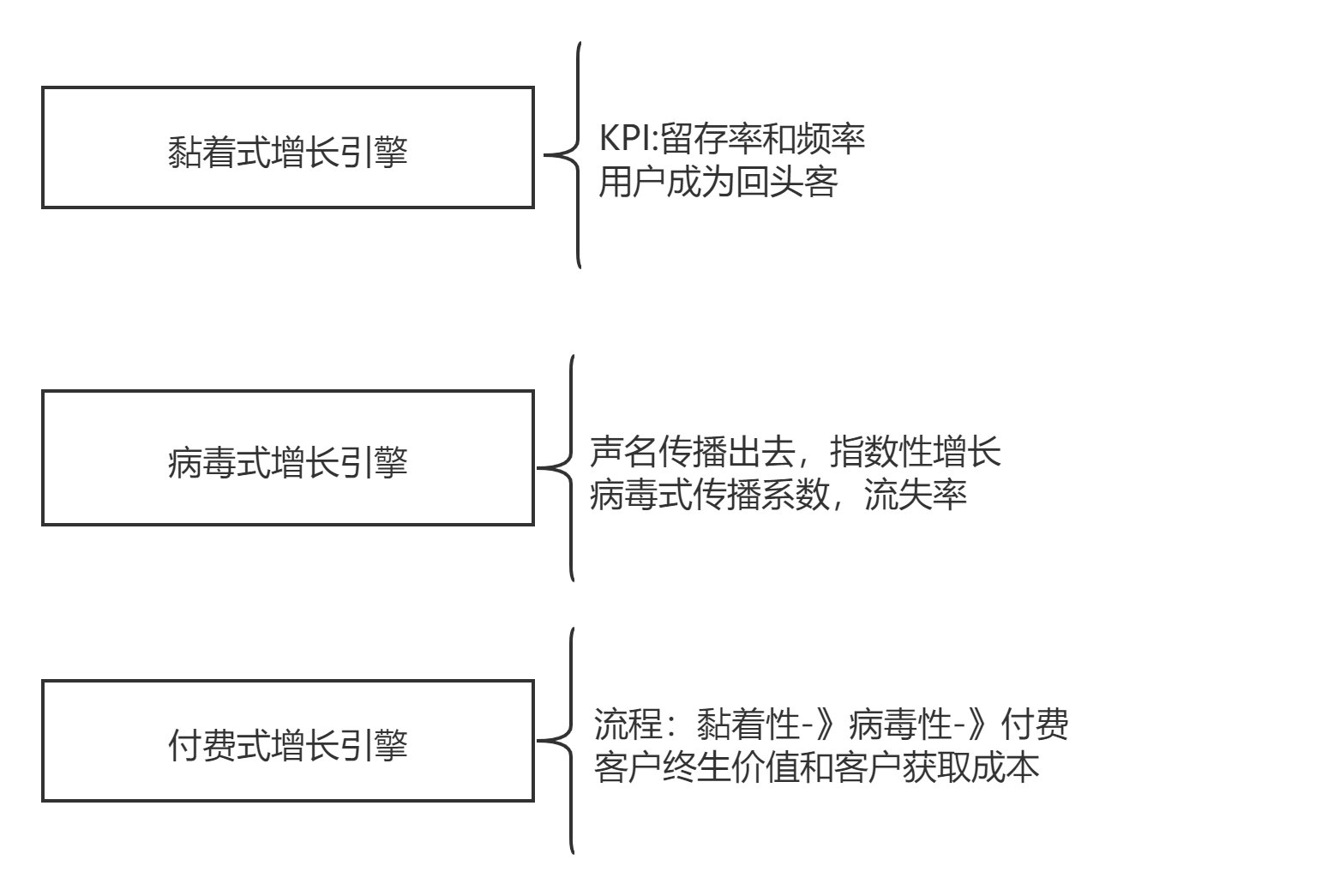### 3.4 第一关键指标

• 解释现阶段最重要的问题。

• 促使得出初始基线并建立清晰的目标。

• 抽象出整个公司层面的健康。

• 不断假设，测量，认知循环。

### 3.5 商业模式

《精益数据分析》中提到六种商业模式。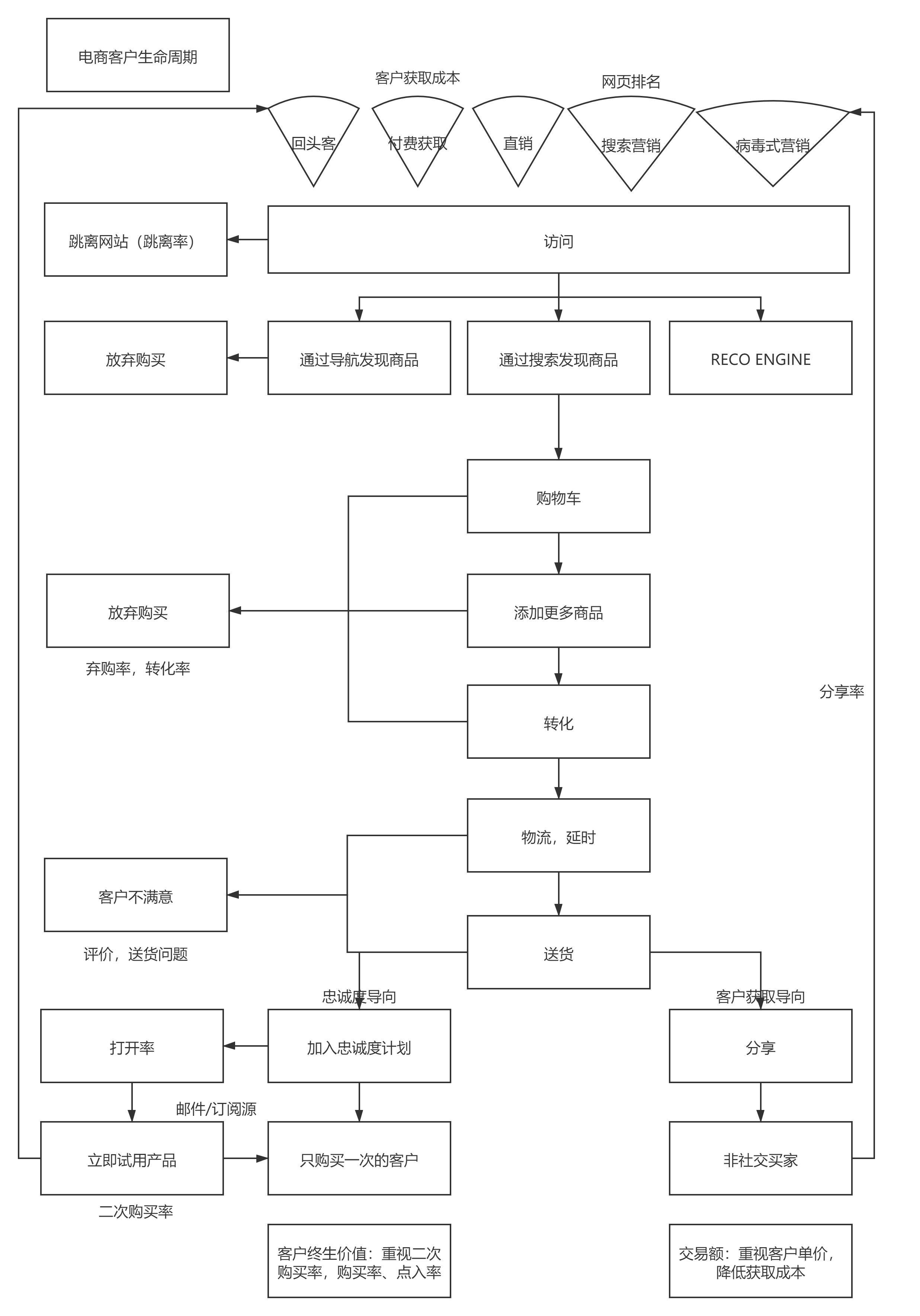SaaS

SaaS指按需提供软件的公司，通常以网站的形式出现。比如一些云平台，软件供应商等等。

SaaS流程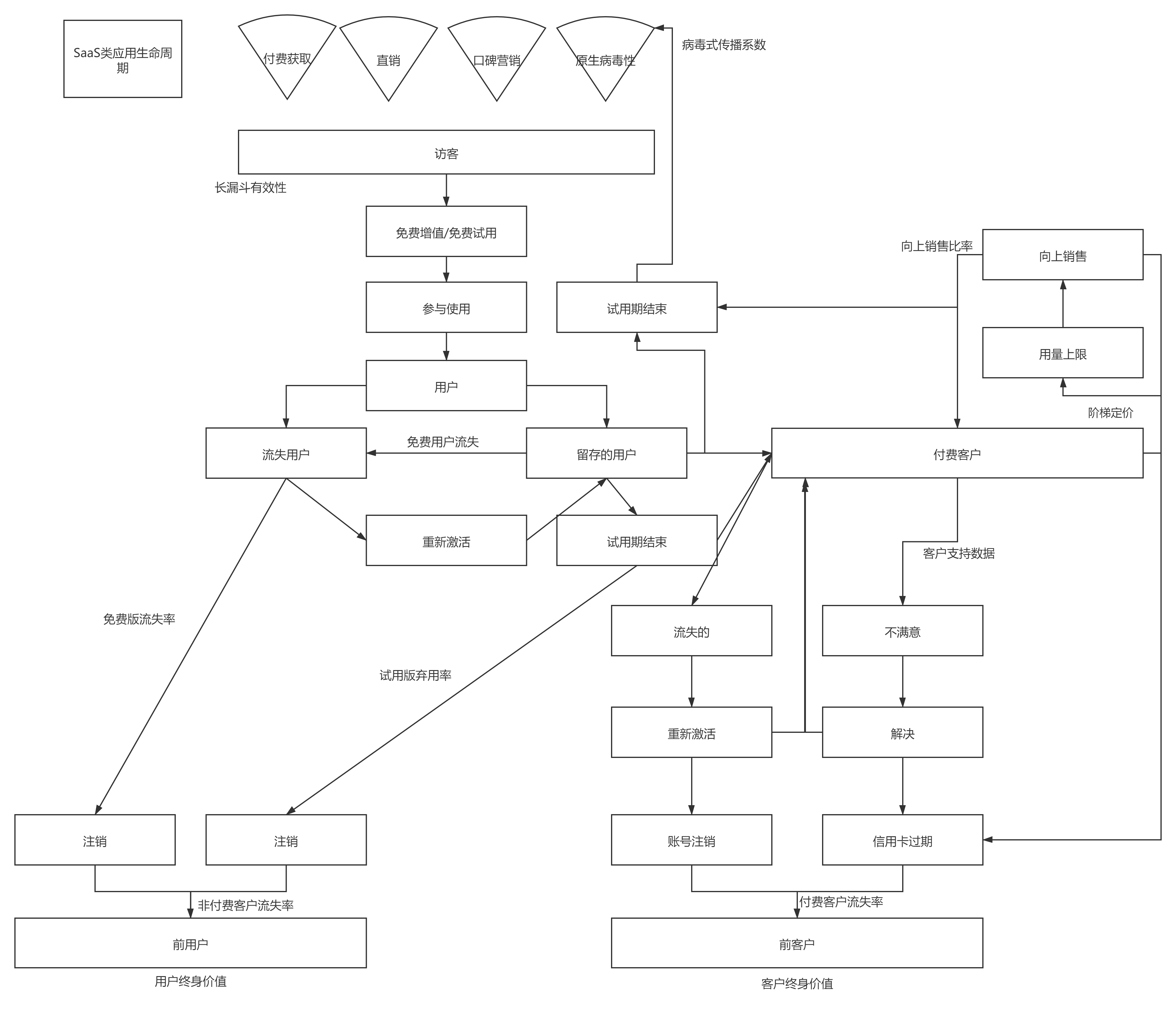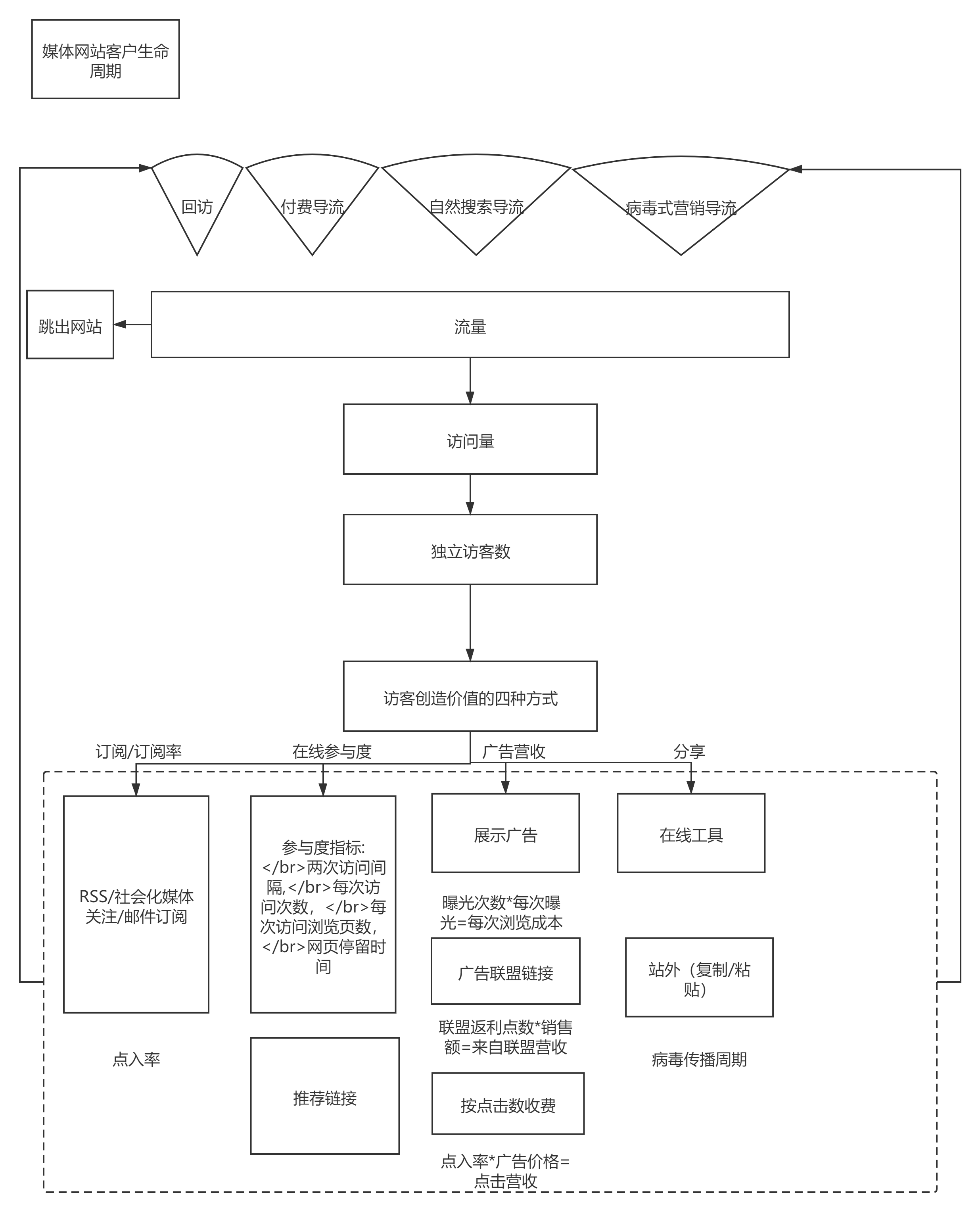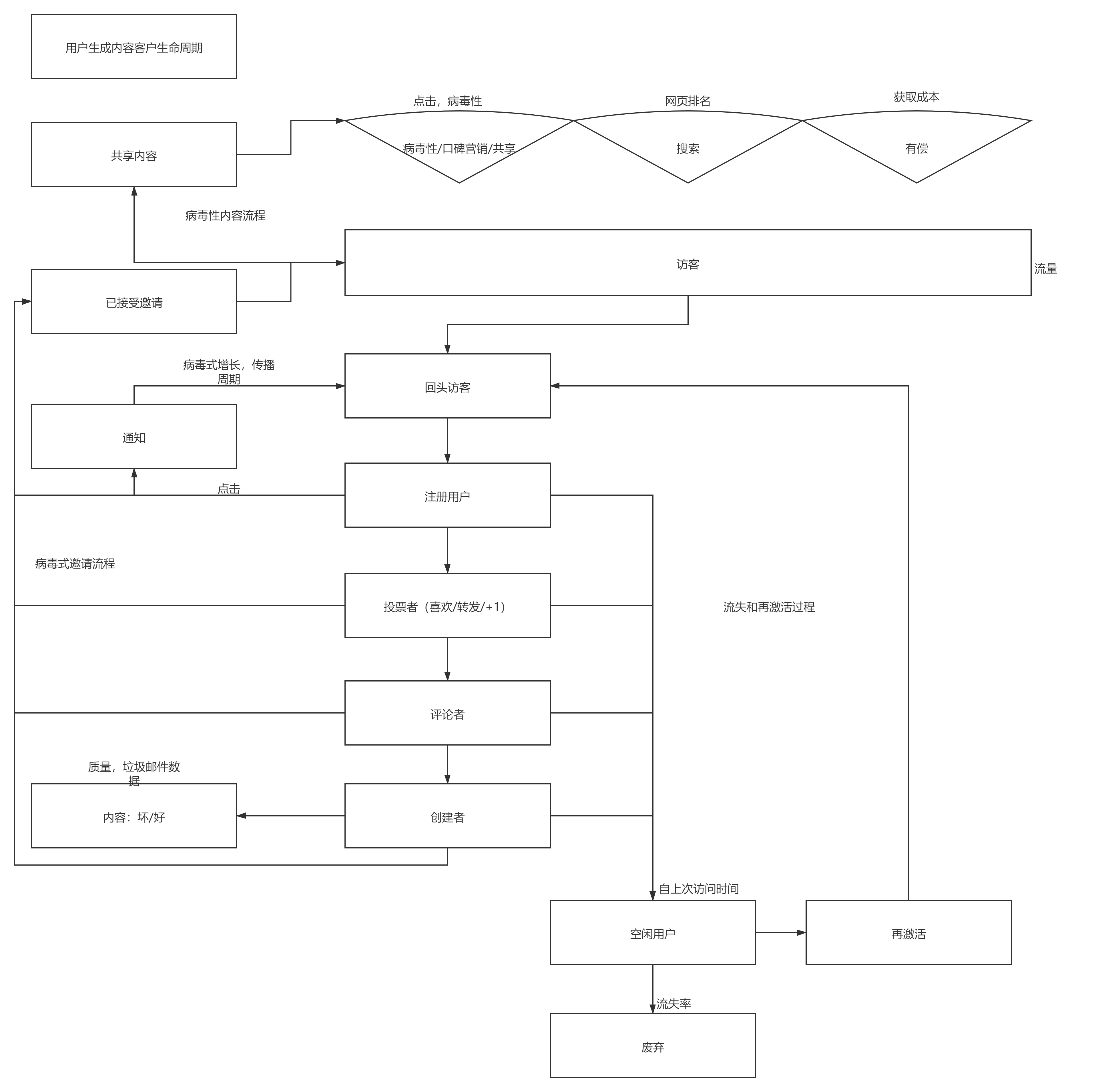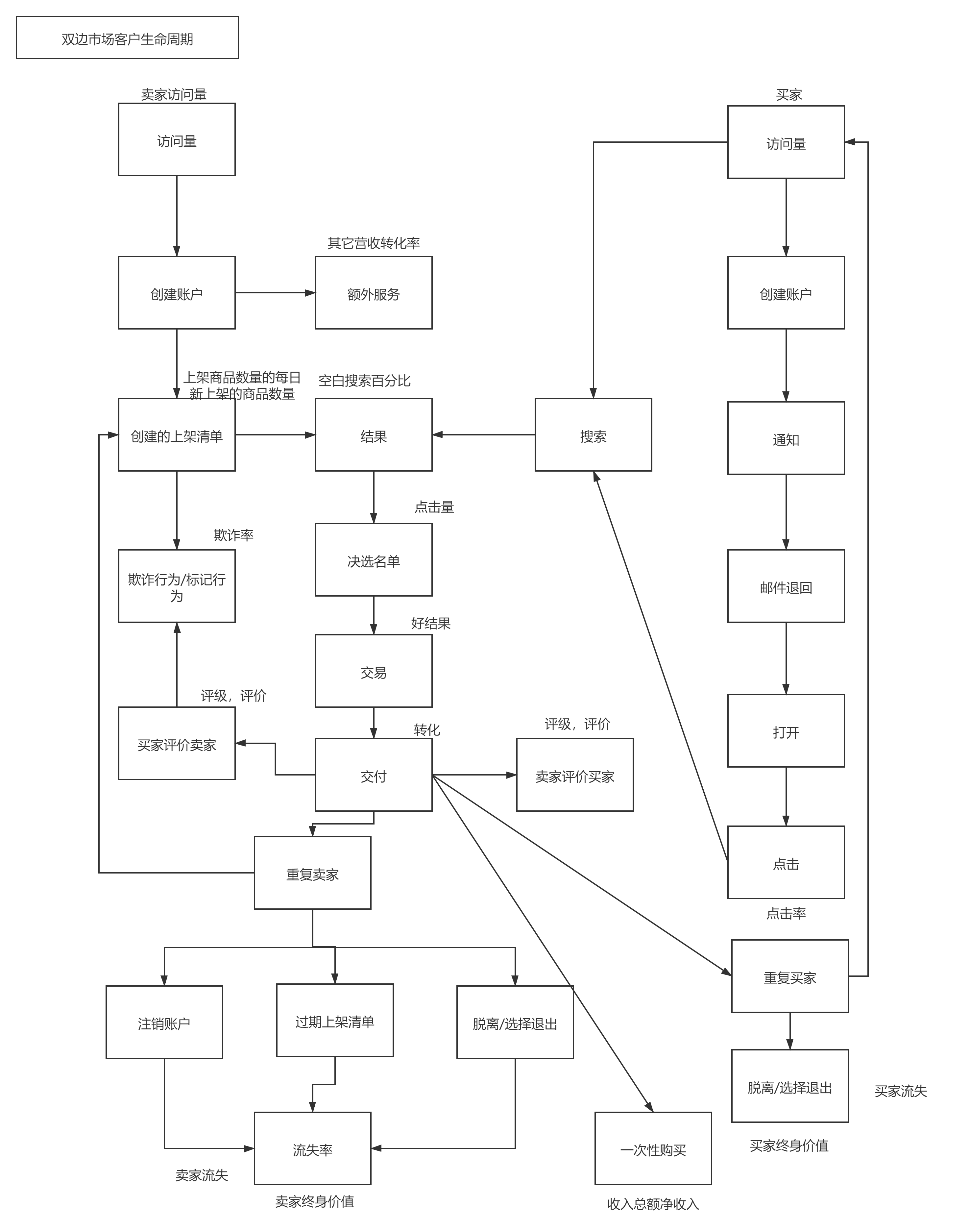## 四.用户演进的阶段

• 移情：你需要深入目标市场，着手解决人们关心的问题，从而促使消费者愿意为你的商品买单。可以用漏斗模型，看模式转化率，例如管理员进入，题库-》试卷-》考试转化率，调查问卷等等

• 黏性：你需要了解自己能否找到已发现问题的解决方案。如果产品糟糕到访客一看到就会厌恶地离开的程度，那么再大力的推广也是毫无意义的。

• 病毒性：在保证产品或服务的黏性后，即可开始口碑营销。

• 营收：该阶段应着手盈利事宜，但并不意味着此前不存在收费行为。

• 规模化：盈利后，公司即可从自身发展模式切换至市场扩张模式。多级分销，地推。

## 五.底线

• 增长率

• 留存率

• 获客成本

• 病毒性

• 可用性和可靠性

### 5.3 获客成本

（客户收入*客户生命周期）— 获客和维护成本

HubSpot 用的计算公式是：

David Skok 的算法是：

（每个用户的平均 MRR *毛利率）/收入流失率

## 六.基础平台建设

### 6.2 开辟期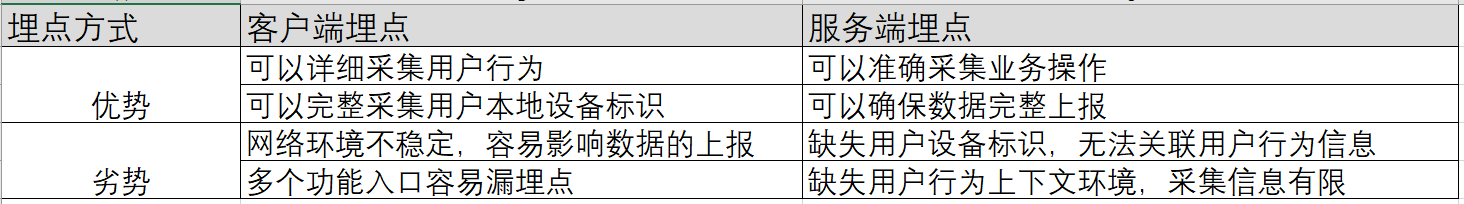## 七.架构体系

### 7.1 架构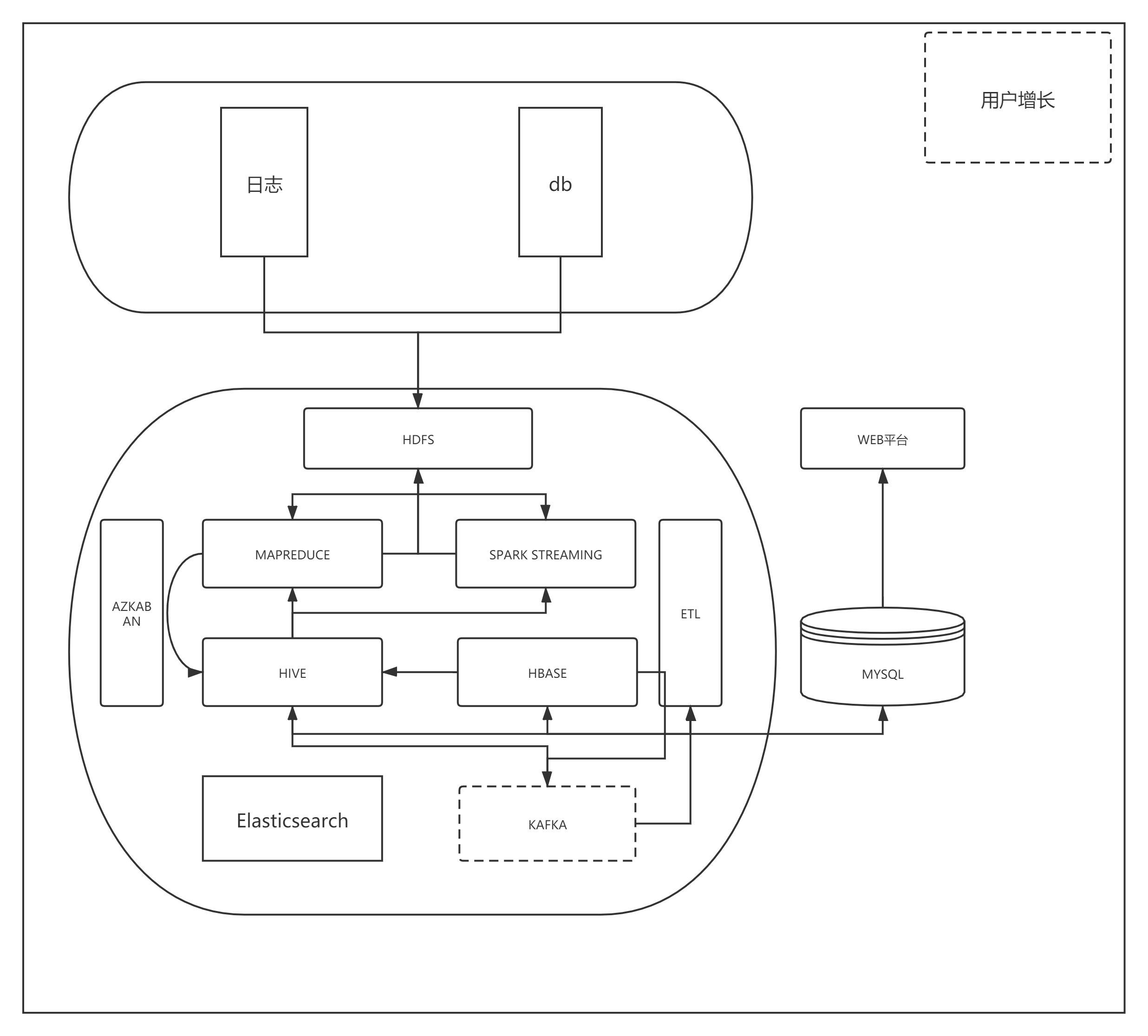• 数据源中日志，服务输出日志，Nginx等等，通过ELK传输出来，一部分从库处理业务数据副本。

• 数据仓库，用的星型模式，维度方面，科室，医院，角色，日期。存储在Hive。

• 计算引擎Spark

• 任务调度Azkaban

• 实时数据Kafka，Elasticsearch，Hbase。

• Lambda架构，这个在以前的Beam文章有介绍，不再赘述。

### 7.2 数据交换

object Data {  abstract class Data  abstract class tables  /**    * hive    * @param warehouseLocation 数据存储位置    * @param dbName 数据库名称    * @param tableName 表名称    * @param year 年    * @param month 月    * @param day 日    */  case class Hive1(warehouseLocation:String,dbName:String,tableName:String,year:String,month:String,day:String)  /**    * hive    * @param warehouseLocation 数据位置    */  case class Hive2(warehouseLocation:String,dbName:String,tableName:String) extends Data  /**    * hive    * @param warehouseLocation 数据位置    */  case class Hive3(warehouseLocation:String,sparkSession:SparkSession) extends Data  /**    * mysql    * @param url 路径带用户密码库名称    * @param sql sql    */  case class MySql1(driver:String,url:String,tableName:String,sql:String) extends Data  case class MySqlByTable(tableName:String,columns: String,values:Array[Row]) extends tables  /**    * mysql    * @param url url    * @param tableName 表名    */  case class MySql2(driver:String,url:String,tableName:String) extends Data   def callCase(f:Data): Any =f match {    case Hive3(a,b)=>      //SparkSession      val appName:String="export_"+TimeUtils.getCertainDayTime(0)      val sparkSession:SparkSession = SparkUtil.hiveSpark(appName,a)      Hive3(a,sparkSession)    case MySql1(a,b,c,d)=>SparkUtil.relationDatabase(a,b)    case MySql2(a,b,c)=>SparkUtil.relationDatabase(a,b

• 操作路由（导入/导出）

• 配置信息

object HiveUtil {  /**    * 禁止严格模式 查询    * @param sqlContext    * @param sqlModel sql    */    def queryByHive(sqlContext:SQLContext,sqlModel:SqlModel2): DataFrame ={      sqlContext.sql(s"USE ${sqlModel.db}") sqlContext.sql("SET hive.mapred.mode=nonstrict") sqlContext.sql(s"select * from${sqlModel.tableName}").drop("year","month","day")    }  /**    * 查询分区表    * @param sqlContext    * @param sqlModel sql    */  def queryByHiveToPartition(sqlContext:SQLContext,sqlModel:SqlModel1): DataFrame ={    sqlContext.sql(s"USE ${sqlModel.db}") sqlContext.sql("SET hive.exec.dynamic.partition=true") sqlContext.sql("SET hive.exec.dynamic.partition.mode=nonstrict") val sqlStringBuilder:StringBuilder=new StringBuilder sqlStringBuilder.append(s"select * from${sqlModel.tableName} where year=${sqlModel.year} and month=${sqlModel.month} ")    if(sqlModel.day.nonEmpty){      sqlStringBuilder.append(s" and day=${sqlModel.day}") sqlContext.sql(sqlStringBuilder.toString()).drop("year","month","day") }else{ sqlContext.sql(sqlStringBuilder.toString()).drop("year","month") } } /** * 插入hive,分区 * @param sql * @param sqlModel * @param view */ def addHive(sql:SQLContext,sqlModel:SqlModel1,view:String): Unit ={ val year=sqlModel.year val month=sqlModel.month val day=sqlModel.day sql.sql(s"USE${sqlModel.db}")    sql.sql("SET hive.exec.dynamic.partition=true")    sql.sql("SET hive.exec.dynamic.partition.mode=nonstrict")    sql.sql(s"INSERT OVERWRITE TABLE ${sqlModel.tableName} PARTITION (year ='$year',month ='$month',day ='$day') SELECT * FROM $view") } /** * 全量插入hive * @param sql * @param sqlModel * @param view */ def addHive(sql:SQLContext,sqlModel:SqlModel2,view:String): Unit ={ sql.sql(s"USE${sqlModel.db}")    sql.sql("SET hive.exec.dynamic.partition=true")    sql.sql("SET hive.exec.dynamic.partition.mode=nonstrict")    sql.sql(s"INSERT OVERWRITE TABLE ${sqlModel.tableName} SELECT * FROM$view")  }  /**    * 查看表详情    */  def tableDetails(sql:SQLContext,sqlModel:SqlModel2): Array[Row] ={    sql.sql(s"USE ${sqlModel.db}") sql.sql("SET hive.exec.dynamic.partition=true") sql.sql("SET hive.exec.dynamic.partition.mode=nonstrict") sql.sql(s"desc${sqlModel.tableName}").collect()  }  /**    * 转换数据    * @param desc hive表详情    * @param dataList mysql数据map集合    * @param sql    * @param view    */  def changeData(desc:Array[Row], dataList:List[Map[String, Any]], sql:SQLContext, view:String): Unit ={    val fields=desc.filter(f=>FilterUtils.isValidatePartitionLine(f.getString(0)))    val fields2=fields.map(x=>{ DataTypes.createStructField(x.getString(0), DataTypes.StringType, false)})    val newData = new util.ArrayList[Row]    //安照hive数据顺序    dataList.foreach(f1 => {      val value=fields.map(f2 => {        var rows:String="null"        val columnName = f2.getString(0)        if (f1.get(columnName).mkString.isEmpty) {            rows ="null"        } else {            rows =f1.get(columnName).mkString        }        rows      })      newData.add(Row.fromSeq(value))    })    sql.createDataFrame(newData,DataTypes.createStructType(fields2)).createOrReplaceTempView(view)  }}case class SqlModel1(db:String,tableName:String,year:String,month:String,day:String)case class SqlModel2(db:String,tabl

MySQL导入到Hive

object ToHive {  val logger: Logger = LoggerFactory.getLogger(ToHive.getClass)  /**    * 全量导入hive    * @param hive    * @param mySql    */  def add(hive:Hive2,mySql:MySql1): Unit ={    Data.callCase(mySql)    println("全量:hive"+hive+" mySql:"+mySql)    val hiveSpark:SparkSession=Data.callCase(Hive3(hive.warehouseLocation,null)).asInstanceOf[Hive3].sparkSession    val hiveSql=hiveSpark.sqlContext    import hiveSql.implicits._    import hiveSpark.implicits._    //mysql视图    val datas=MySqlUtils.queryAll(mySql.sql)    val desc=HiveUtil.tableDetails(hiveSql,SqlModel2(hive.dbName,hive.tableName))    //清洗数据    HiveUtil.changeData(desc,datas,hiveSql,"model")    HiveUtil.addHive(hiveSql,SqlModel2(hive.dbName,hive.tableName),"model")    ConnectionPool.closeAll    hiveSpark.stop()  }  /**    *    * hive分区批量增加    * @param hive    * @param mySql    */  def add(hive:Hive1,mySql:MySql1): Unit ={    Data.callCase(mySql)    println("分区增量:hive"+hive+" mySql:"+mySql)    val hiveSpark:SparkSession=Data.callCase(Hive3(hive.warehouseLocation,null)).asInstanceOf[Hive3].sparkSession    val hiveSql=hiveSpark.sqlContext    import hiveSpark.implicits._    import hiveSql.implicits._    //mysql视图    val datas=MySqlUtils.queryAll(mySql.sql)    val desc=HiveUtil.tableDetails(hiveSql,SqlModel2(hive.dbName,hive.tableName))    HiveUtil.changeData(desc,datas,hiveSql,"model")    HiveUtil.addHive(hiveSql,SqlModel1(hive.dbName,hive.tableName,hive.year,hive.month,hive.day),"model")    ConnectionPool.closeAll    hiveSpark.st

Hive导出

object ToRelation {  val logger: Logger = LoggerFactory.getLogger(ToRelation.getClass)  /**    * 增量    * @param hive    * @param mySql    */    def incrementByTime(hive:Hive1,mySql:MySql2): Unit ={      Data.callCase(mySql)      println("incrementByTime:hive"+hive+" mySql:"+mySql)      val hiveSpark:SparkSession=Data.callCase(Hive3(hive.warehouseLocation,null)).asInstanceOf[Hive3].sparkSession      val hiveSql=hiveSpark.sqlContext      val hiveDbName=hive.dbName      val hiveTableName=hive.tableName      val mySqlTableName=mySql.tableName      val hivedF=HiveUtil.queryByHiveToPartition(hiveSql,SqlModel1(hiveDbName,hiveTableName,hive.year,hive.month,hive.day))      val columns=hivedF.columns.filter(f=>FilterUtils.isValidatePartitionLine(f.toString)).mkString(",")      MySqlUtils.batchSave(MySqlByTable(mySqlTableName,columns,hivedF.collect()))      logger.info("incrementByTime:hive"+hive+" mySql:"+mySql)      ConnectionPool.closeAll      hiveSpark.stop()    }  /**    * 批量增加所有数据    * @param hive    * @param mySql    */    def add(hive:Hive2,mySql:MySql2): Unit ={      Data.callCase(mySql)      println("add:hive"+hive+" mySql:"+mySql)      val hiveSpark:SparkSession=Data.callCase(Hive3(hive.warehouseLocation,null)).asInstanceOf[Hive3].sparkSession      val hiveSql=hiveSpark.sqlContext      val hiveDbName=hive.dbName      val hiveTableName=hive.tableName      val mySqlTableName=mySql.tableName      val hivedF=HiveUtil.queryByHive(hiveSql,SqlModel2(hiveDbName,hiveTableName))      val columns=hivedF.columns.filter(f=>FilterUtils.isValidatePartitionLine(f.toString)).mkString(",")      MySqlUtils.batchSave(MySqlByTable(mySqlTableName,columns,hivedF.collect()))      logger.info("add:hive"+hive+" mySql:"+mySql)      ConnectionPool.closeAll      hiveSpark.stop()

### 7.3 数据仓库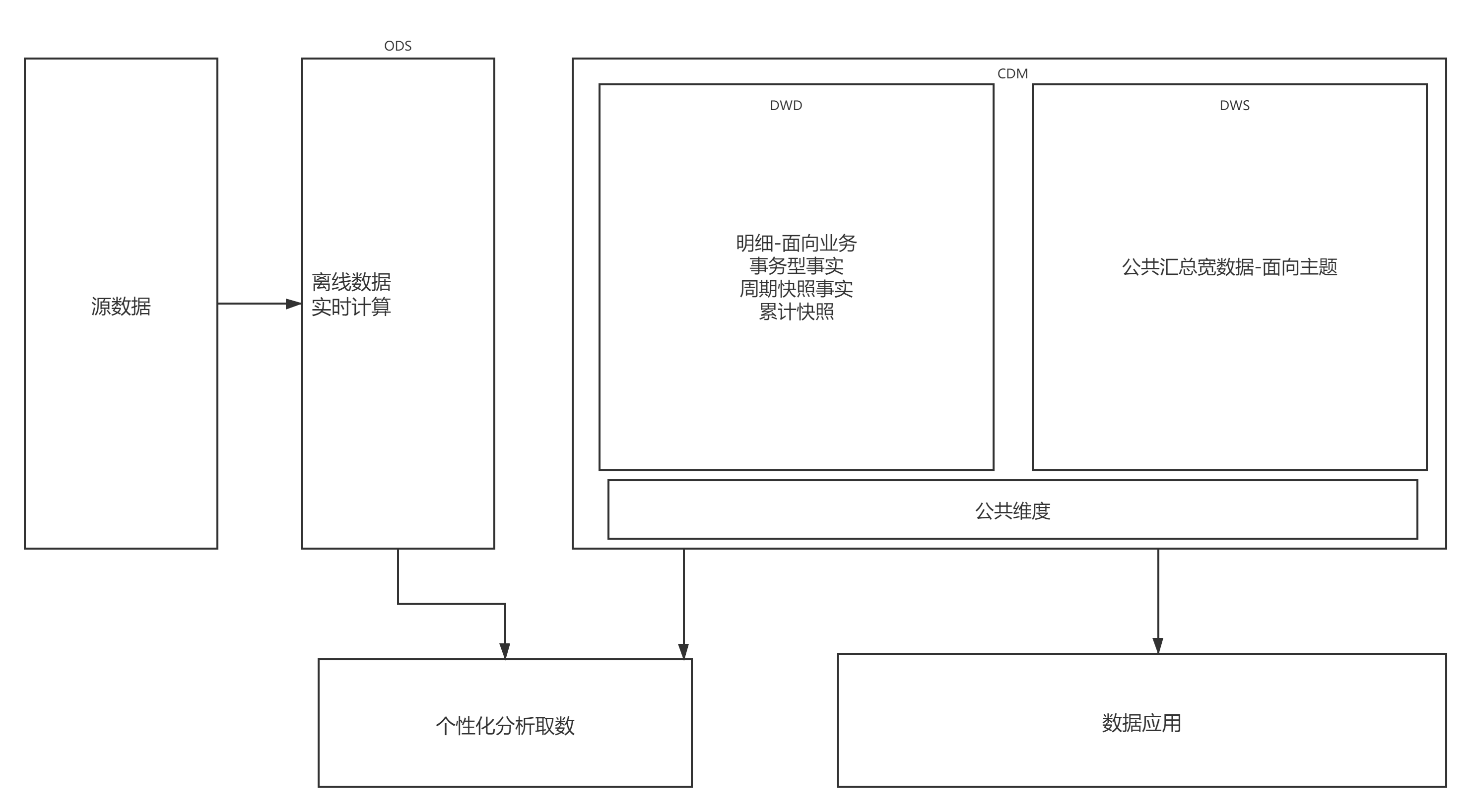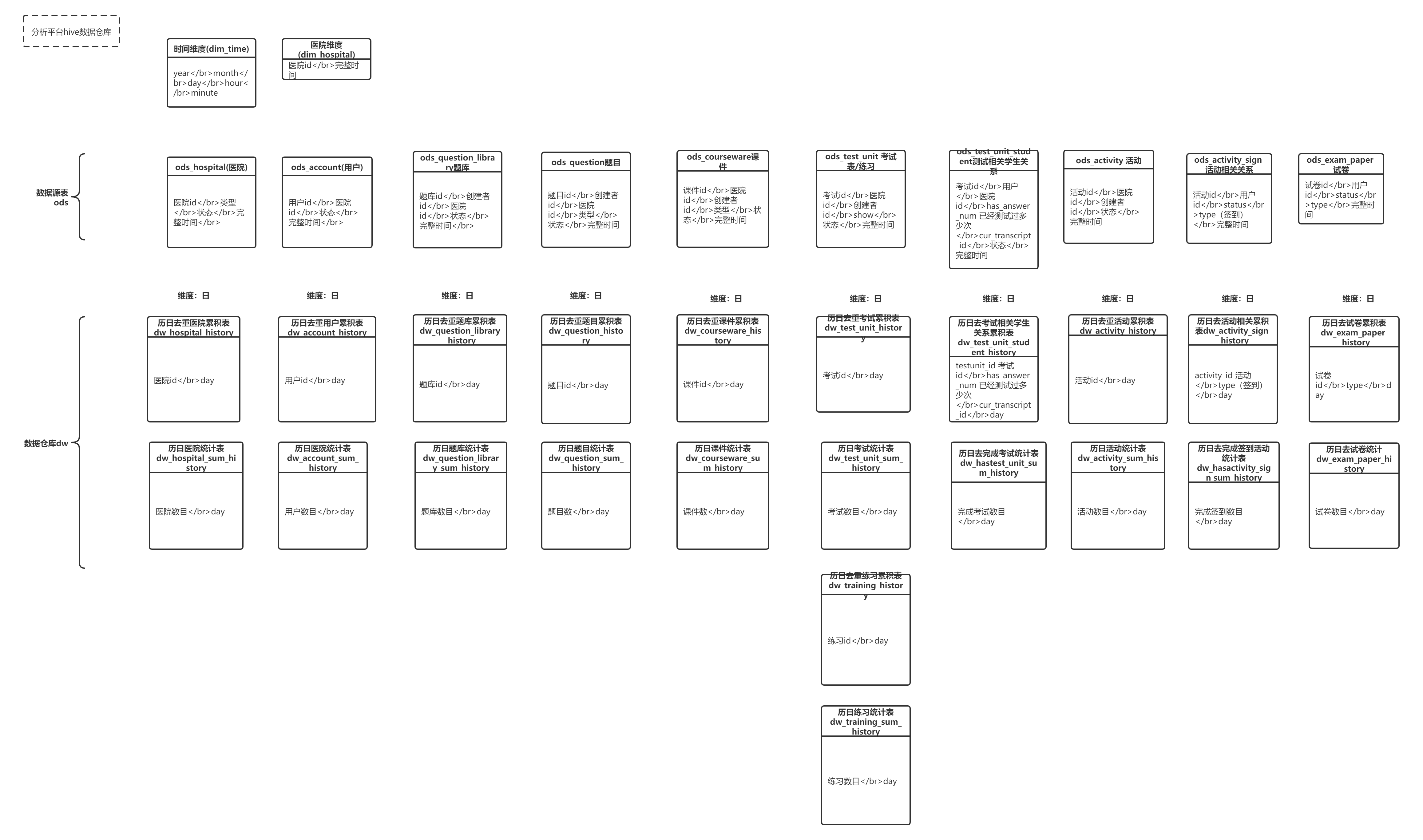### 7.4 任务处理流程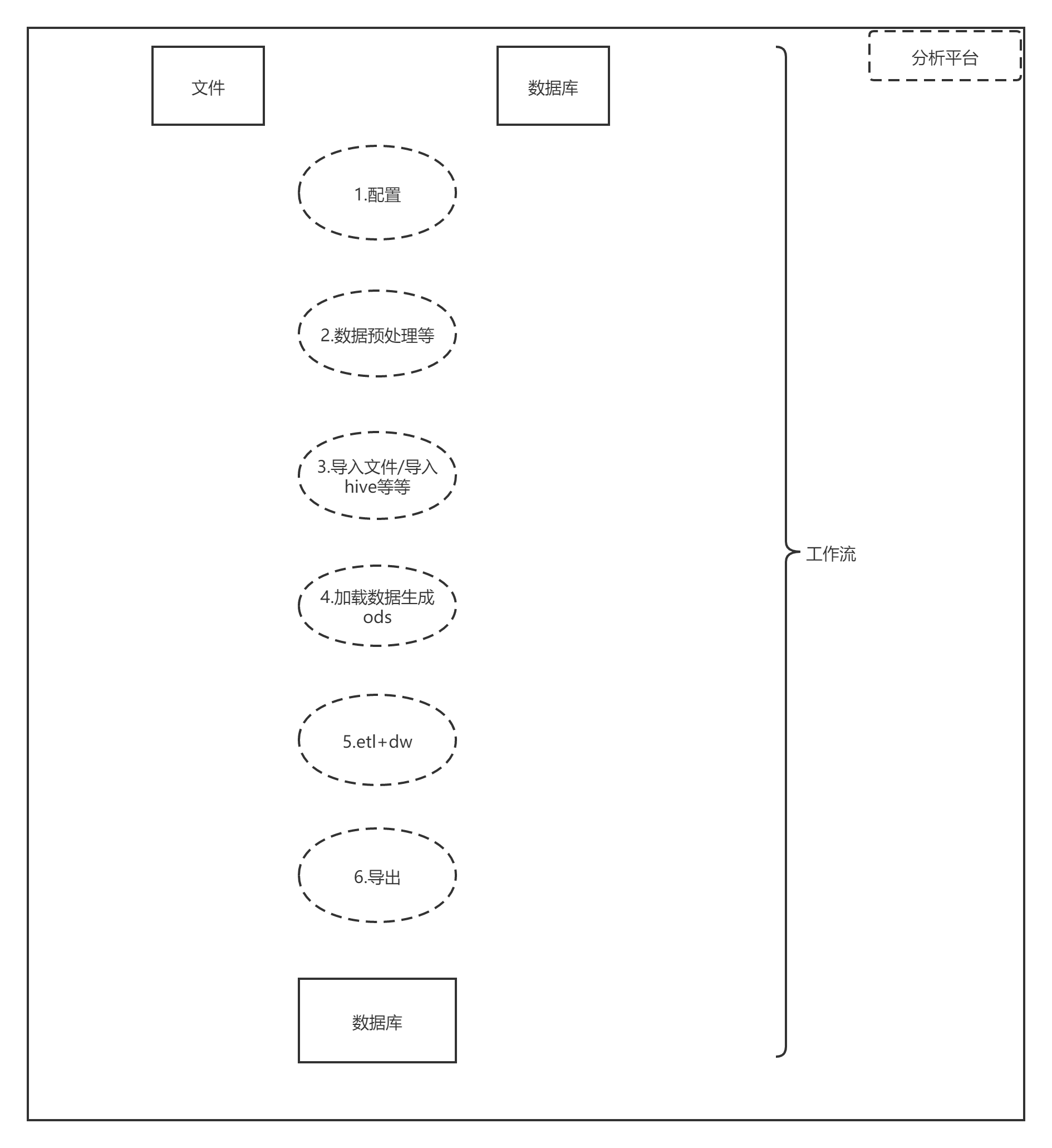• 这个流程做好自动化，因为这种任务很多。

• 收集日志，为后续异常处理。

• 监控对象

• 监控那些指标

• 哪些维度监控

## 八.基础指标

/**  * 127.0.0.1 - - [06/Jun/2018:00:00:00 +0800] "GET /icu-system/mobile/courseware/query/onlinecourseware/list?page=3&pagesize=10&session_id=f600b1750c2c1ffde0ae23728d7eff6d7b40f8385c289353d4d5027e6a36c48c HTTP/1.0" 200 1495  *  * @author limeng  *   **/object AccessLog {  val logger = LoggerFactory.getLogger(classOf[AccessLog])  //日志正则  val PARTTERN: Regex =    """^(\S+) (\S+) (\S+) $([\w:/]+\s[+\-]\d{4})$ "(\S+) (\S+) (\S+)" (\d{3}) (\d+)""".r  /**    * 验证数据是否符合格式    */  def isValidateLogLine(line: String): Boolean = {    val options = PARTTERN.findFirstMatchIn(line)    if(options.isEmpty){      false    }else{      true    }  }  /**    * 解析输入日志数据    */  def parseLogLine(line:String):AccessLog={    if(!isValidateLogLine(line)){      logger.error("参数格式异常")      throw new IllegalArgumentException("参数格式异常")    }    // 从line中获取匹配的数据    val options = PARTTERN.findFirstMatchIn(line)    val matcher=options.get    // 构建返回值    AccessLog(      matcher.group(1), // 获取匹配字符串中第一个小括号中的值      matcher.group(2),      matcher.group(3),      matcher.group(4),      matcher.group(5),      matcher.group(6),      matcher.group(7),      matcher.group(8),      matcher.group(9)    )  }}case class AccessLog(ipAddress: String, // IP地址                     clientId: String, // 客户端唯一标识符                     userId: String, // 用户唯一标识符                     serverTime: String, // 服务器时间                     method: String, // 请求类型/方式                     endpoint: String, // 请求的资源                     protocol: String, // 请求的协议名称                     responseCode: String, // 请求返回值：比如：200、401                     contentSize: String // 返回的结果数据大小                    )                 object AccessCutting {    def cuttingFile(accessFile:String): Unit ={        val hiveSpark=SparkUtil.hiveSpark("AccessCutting")        val sc=hiveSpark.sparkContext        val path=accessFile        val fileRdd=sc.textFile(path)        val fileName=path.split("/").last.filter(f=>StringUtils.isSymbol(f.toString))        //过滤数据，对数据转换操作        val accessLog=fileRdd.filter(line=>AccessLog.isValidateLogLine(line))          .map(line=>{              AccessLog.parseLogLine(line)          })        accessLog.cache()        val dataFrame=hiveSpark.createDataFrame(accessLog)        dataFrame.createOrReplaceTempView("url_access")        //年月日        //val yearMonthDay=TimeUtils.getYearMonthDay(-1)        val fileDate=TimeUtils(TimeUtils.strToDate(fileName))        val fileYearMonthDay=TimeUtils.getYearMonthDay(0)        val year=fileYearMonthDay.year        val month=fileYearMonthDay.month        val day=fileYearMonthDay.day        hiveSpark.sql("USE hushijie_dw")        hiveSpark.sql("SET hive.exec.dynamic.partition=true")        hiveSpark.sql("SET hive.exec.dynamic.partition.mode=nonstrict")        hiveSpark.sql(s"INSERT OVERWRITE TABLE ods_url_access PARTITION (year ='$year',month ='$month',day ='$day') SELECT * FROM url_access") accessLog.unpersist() sc.stop() hiveSp 医院活跃度 import com.icu.bigdata.model.NewOldUsersimport com.icu.bigdata.utils._import org.slf4j.LoggerFactoryimport scalikejdbc._import scala.collection.mutable.ListBufferobject Hospital { val logger = LoggerFactory.getLogger(Hospital.getClass) /** * 增加活跃度 */ def addActivity(): Unit ={ val hiveSpark=SparkUtil.hiveSpark("addActivity") val sql=hiveSpark.sqlContext import sql.implicits._ val fileYearMonthDay=TimeUtils.getYearMonthDay(-1) val year=fileYearMonthDay.year val month=fileYearMonthDay.month val day=fileYearMonthDay.day sql.sql("USE hushijie_dw") sql.sql(s"select * from dw_method_total where year ='$year' and month ='$month' and day ='$day' " +      s"and methodname in('appLogin','hospitalLogin') limit 10").createOrReplaceTempView("methods")    //sql.sql(s"select * from dw_method_total where year ='$year' and month ='$month' and day ='$day' " + // s"and methodname ='doFilter' and classname='c.i.f.HttpRequestCharacterFilter' ").createOrReplaceTempView("methods") sql.cacheTable("methods") //获取用户id集合，去除null val accountids=sql.sql("select accountids from methods").collect().flatMap(x=>{ x.mkString.split(",").filter(line=>NewOldUsers.isValidateAccountids(line)) }) sql.uncacheTable("methods") MysqlConnectionUtils.formMysql1() var activity=new ListBuffer[Int] //取出医院id accountids.foreach(t=>{ val id=t.toInt //println(id) val activitySql="select a.hospital from account a join hospital h on a.hospital=h.id " + " where 1=1 and a.id="+id+" and h.level<>13" val value = DB.readOnly(implicit session => SQL(activitySql).map({ rs => rs.intOpt("hospital").getOrElse(0) }).list().apply().mkString) if(!value.isEmpty){ activity.append(value.toInt) } }) val newDate=TimeUtils.getCertainDayTimeStr(0) val beforeDate=TimeUtils.getCertainDayTimeStr(-1) val totalSql=s"""select count(1) as num from hospital where 1=1 and to_days(ctime)<to_days('%s') and level<>13""".format(newDate) //获取当前医院总数 val total=DB.readOnly(implicit session => SQL(totalSql).map({ rs => rs.int("num") }).list().apply().mkString) ConnectionPool.closeAll //活跃医院总数 val currTotal=activity.distinct.size sql.createDataset(Seq((total,currTotal.toString,beforeDate))).createOrReplaceTempView("activitys") val sqlModel=SqlModel("hushijie_dw","dw_hospital_activity","activitys") SqlUtil.addHive(sql,sqlModel,fileYearMonthDay) logger.info("成功----"+newDate) hiveSpar 统计所有方法中间表 /** * 统计所有方法 */def addMethodTotal(): Unit ={ val hiveSpark=SparkUtil.hiveSpark("addMethodTotal") import hiveSpark.implicits._ val sql=hiveSpark.sqlContext import sql.implicits._ val fileYearMonthDay=TimeUtils.getYearMonthDay(-1) val year=fileYearMonthDay.year val month=fileYearMonthDay.month val day=fileYearMonthDay.day sql.sql("USE hushijie_dw") sql.sql("set hive.fetch.task.conversion=none") sql.sql("set hive.exec.orc.split.strategy=BI") sql.sql(s"select classname,methodname,accountid,requestid,result" + s" from ods_url_logstash where year ='$year' and  month ='$month' and day ='$day'").createOrReplaceTempView("logs")  sql.cacheTable("logs")  sql.sql("select  classname,methodname,concat_ws(',',collect_set(accountid)) as accountids," +    "concat_ws(',',collect_set(requestid)) as requestids, concat_ws(',',collect_set(result)) as results " +    "from logs  group by classname,methodname ").createOrReplaceTempView("methods")  sql.uncacheTable("logs")  val sqlModel=SqlModel("hushijie_dw","dw_method_total","methods")  SqlUtil.addHive(sql,sqlModel,fileYearMonthDay)  logger.info("addMethodTotal成功-----")  hiveSpark

## 九.闭环体系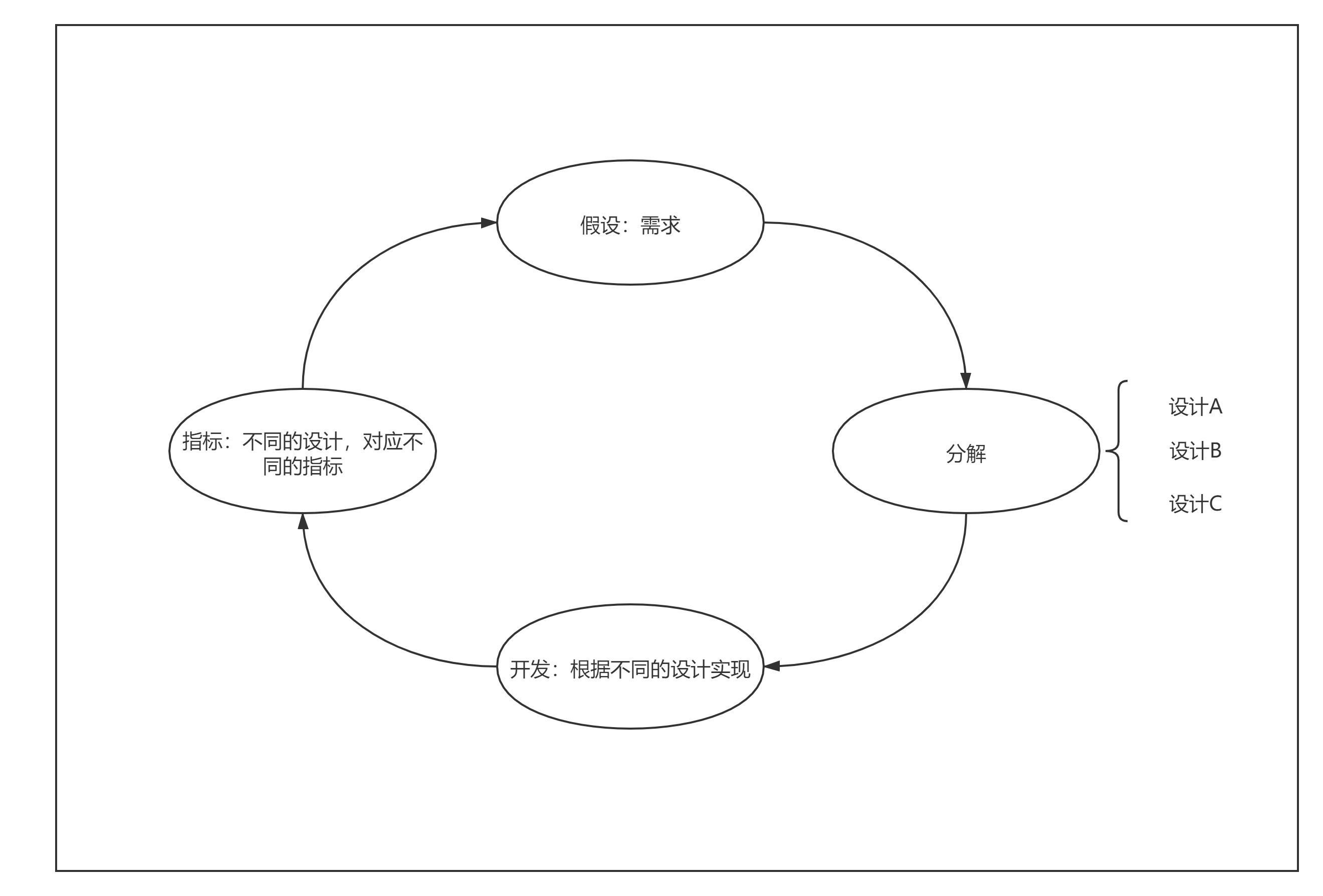## 十.用户分级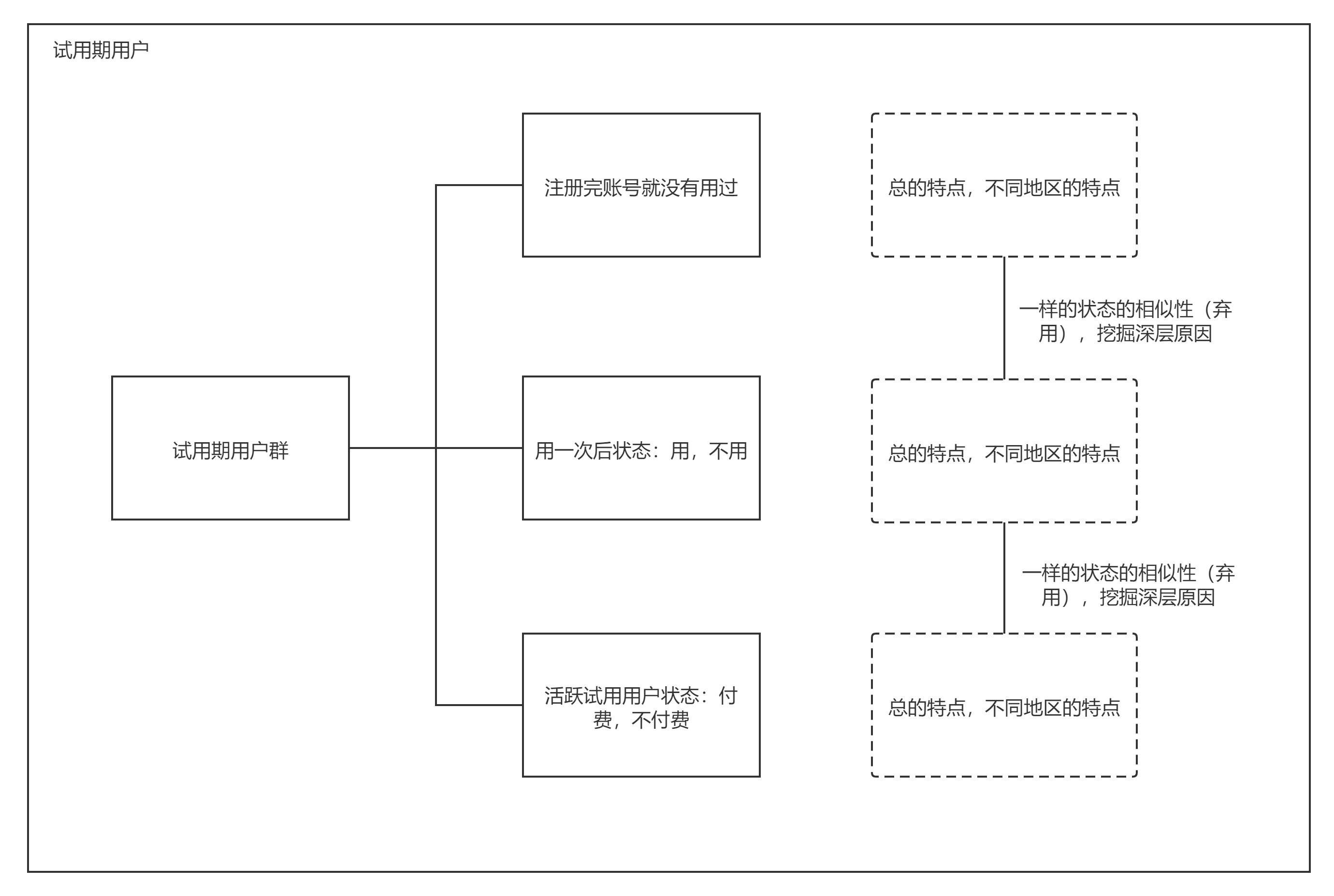## 十.一些周边应用

• 因为产品涉及到考试，会有作弊情况，通过爬虫，测试库，可以去比对考试题情况，这个方式一般两种，一种系统端，做些策略，一种业务上做些保护措施，比如加密，通过用户的一些成绩详细信息列入可疑范围名单。

• 题库去重，当时的策略，海明距离。

• 预测收益，行为。

• 标签库。

• 分词

• hash

• 加权

• 合并

• 降维

• 拆分

• 比对

public class Simhash implements Serializable {    private static final long serialVersionUID = 4117064662596213588L;    private JiebaSegmenter segmenter = new JiebaSegmenterChild(); // 分词 名词动词形容词    private List<Map<String, List<Long>>> storage = new ArrayList<Map<String, List<Long>>>();// 按照分段存储simhash，查找更快速    private int bitNum = 64;    private int fracCount = 4; // 默认按照4段进行simhash存储    private int fracBitNum = bitNum / fracCount;//分组16位为一组    private int hammingThresh = 3;// 汉明距离的衡量标准    public Simhash() {        for (int i = 0; i < fracCount; i++)            storage.add(new HashMap<String, List<Long>>());    }    public Simhash(int fracCount, int hammingThresh) {        this.fracCount = fracCount;        fracBitNum = bitNum / fracCount;        this.hammingThresh = hammingThresh;        for (int i = 0; i < fracCount; i++)            storage.add(new HashMap<String, List<Long>>());    }    /**     * 指定文本计算simhash值     *     * @param content     * @return Long     */    public Long calSimhash(String content) {        // p{Punct} 标点符号：!"#\$%&'()*+,-./:;<=>?@[\]^_{|}~        // p{Space} 空白字符：[ \t\n\x0B\f\r]        String filterContent = content.trim().replaceAll("\\p{Punct}|\\p{Space}", "");        // 切词        List<SegToken> lsegStr = segmenter.process(filterContent, JiebaSegmenter.SegMode.SEARCH);        // 按照词语的hash值，计算simHashWeight(低位对齐)        Integer[] weight = new Integer[bitNum];        Arrays.fill(weight, 0);        for (SegToken st : lsegStr) {            long wordHash = Murmur3.hash64(st.word.getBytes());            for (int i = 0; i < bitNum; i++) {                if (((wordHash >> i) & 1) == 1) weight[i] += 1;                else weight[i] -= 1;            }        }        // 计算得到Simhash值        StringBuilder sb = new StringBuilder();        for (int i = 0; i < bitNum; i++) {            if (weight[i] > 0) sb.append(1);            else sb.append(0);        }        return new BigInteger(sb.toString(), 2).longValue();    }    /**     * 判断文本是否重复     *     * @param content     * @return     */    public boolean isDuplicate(String content,Long oldSimhash) {        Long simhash=null;        if(oldSimhash == null){            simhash = calSimhash(content);        }else{            simhash = oldSimhash;        }        List<String> lFrac = splitSimhash(simhash);        int dis = 0;        for (int i = 0; i < fracCount; i++) {            String frac = lFrac.get(i);            Map<String, List<Long>> fracMap = storage.get(i);            if (fracMap.containsKey(frac)) {                for (Long simhash2 : fracMap.get(frac)) {                    if (hamming(simhash, simhash2) < hammingThresh) return true;                }            }        }        return false;    }    /**     * 按照(frac, <simhash, content>)索引进行存储     *     * @param simhash     * @param content     */    public void store(Long simhash, String content) {        List<String> lFrac = splitSimhash(simhash);        for (int i = 0; i < fracCount; i++) {            String frac = lFrac.get(i);            Map<String, List<Long>> fracMap = storage.get(i);            if (fracMap.containsKey(frac)) fracMap.get(frac).add(simhash);            else {                List<Long> ls = new ArrayList<Long>();                ls.add(simhash);                fracMap.put(frac, ls);            }        }    }    // 计算汉明距离    private int hamming(Long s1, Long s2) {        int dis = 0;        for (int i = 0; i < bitNum; i++) {            if ((s1 >> i & 1) != (s2 >> i & 1)) dis++;        }        return dis;    }    private int hamming(String s1, String s2) {        if (s1.length() != s2.length()) return 0;        int dis = 0;        for (int i = 0; i < s1.length(); i++) {            if (s1.charAt(i) != s2.charAt(i)) dis++;        }        return dis;    }    // 将simhash分成n段    private List<String> splitSimhash(Long simhash) {        List<String> ls = new ArrayList<String>();        StringBuilder sb = new StringBuilder();        for (int i = 0; i < bitNum; i++) {            sb.append(simhash >> i & 1);            if ((i + 1) % fracBitNum == 0) {                ls.add(sb.toString());                sb.setLength(0);            }        }        return ls;    }}//hash算法public class Murmur3 implements Serializable {    // Constants for 32 bit variant    private static final int C1_32 = 0xcc9e2d51;    private static final int C2_32 = 0x1b873593;    private static final int R1_32 = 15;    private static final int R2_32 = 13;    private static final int M_32 = 5;    private static final int N_32 = 0xe6546b64;    // Constants for 128 bit variant    private static final long C1 = 0x87c37b91114253d5L;    private static final long C2 = 0x4cf5ad432745937fL;    private static final int R1 = 31;    private static final int R2 = 27;    private static final int R3 = 33;    private static final int M = 5;    private static final int N1 = 0x52dce729;    private static final int N2 = 0x38495ab5;    private static final int DEFAULT_SEED = 0;    private static final long serialVersionUID = -6219781538366994038L;    /**     * Murmur3 32-bit variant.     *     * @param data - input byte array     * @return - hashcode     */    public static int hash32(byte[] data) {        return hash32(data, data.length, DEFAULT_SEED);    }    /**     * Murmur3 32-bit variant.     *     * @param data   - input byte array     * @param length - length of array     * @param seed   - seed. (default 0)     * @return - hashcode     */    public static int hash32(byte[] data, int length, int seed) {        int hash = seed;        final int nblocks = length >> 2;        // body        for (int i = 0; i < nblocks; i++) {            int i_4 = i << 2;            int k = (data[i_4] & 0xff)                    | ((data[i_4 + 1] & 0xff) << 8)                    | ((data[i_4 + 2] & 0xff) << 16)                    | ((data[i_4 + 3] & 0xff) << 24);            // mix functions            k *= C1_32;            k = Integer.rotateLeft(k, R1_32);            k *= C2_32;            hash ^= k;            hash = Integer.rotateLeft(hash, R2_32) * M_32 + N_32;        }        // tail        int idx = nblocks << 2;        int k1 = 0;        switch (length - idx) {            case 3:                k1 ^= data[idx + 2] << 16;            case 2:                k1 ^= data[idx + 1] << 8;            case 1:                k1 ^= data[idx];                // mix functions                k1 *= C1_32;                k1 = Integer.rotateLeft(k1, R1_32);                k1 *= C2_32;                hash ^= k1;        }        // finalization        hash ^= length;        hash ^= (hash >>> 16);        hash *= 0x85ebca6b;        hash ^= (hash >>> 13);        hash *= 0xc2b2ae35;        hash ^= (hash >>> 16);        return hash;    }    /**     * Murmur3 64-bit variant. This is essentially MSB 8 bytes of Murmur3 128-bit variant.     *     * @param data - input byte array     * @return - hashcode     */    public static long hash64(byte[] data) {        return hash64(data, data.length, DEFAULT_SEED);    }    /**     * Murmur3 64-bit variant. This is essentially MSB 8 bytes of Murmur3 128-bit variant.     *     * @param data   - input byte array     * @param length - length of array     * @param seed   - seed. (default is 0)     * @return - hashcode     */    public static long hash64(byte[] data, int length, int seed) {        long hash = seed;        final int nblocks = length >> 3;        // body        for (int i = 0; i < nblocks; i++) {            final int i8 = i << 3;            long k = ((long) data[i8] & 0xff)                    | (((long) data[i8 + 1] & 0xff) << 8)                    | (((long) data[i8 + 2] & 0xff) << 16)                    | (((long) data[i8 + 3] & 0xff) << 24)                    | (((long) data[i8 + 4] & 0xff) << 32)                    | (((long) data[i8 + 5] & 0xff) << 40)                    | (((long) data[i8 + 6] & 0xff) << 48)                    | (((long) data[i8 + 7] & 0xff) << 56);            // mix functions            k *= C1;            k = Long.rotateLeft(k, R1);            k *= C2;            hash ^= k;            hash = Long.rotateLeft(hash, R2) * M + N1;        }        // tail        long k1 = 0;        int tailStart = nblocks << 3;        switch (length - tailStart) {            case 7:                k1 ^= ((long) data[tailStart + 6] & 0xff) << 48;            case 6:                k1 ^= ((long) data[tailStart + 5] & 0xff) << 40;            case 5:                k1 ^= ((long) data[tailStart + 4] & 0xff) << 32;            case 4:                k1 ^= ((long) data[tailStart + 3] & 0xff) << 24;            case 3:                k1 ^= ((long) data[tailStart + 2] & 0xff) << 16;            case 2:                k1 ^= ((long) data[tailStart + 1] & 0xff) << 8;            case 1:                k1 ^= ((long) data[tailStart] & 0xff);                k1 *= C1;                k1 = Long.rotateLeft(k1, R1);                k1 *= C2;                hash ^= k1;        }        // finalization        hash ^= length;        hash = fmix64(hash);        return hash;    }    /**     * Murmur3 128-bit variant.     *     * @param data - input byte array     * @return - hashcode (2 longs)     */    public static long[] hash128(byte[] data) {        return hash128(data, data.length, DEFAULT_SEED);    }    /**     * Murmur3 128-bit variant.     *     * @param data   - input byte array     * @param length - length of array     * @param seed   - seed. (default is 0)     * @return - hashcode (2 longs)     */    public static long[] hash128(byte[] data, int length, int seed) {        long h1 = seed;        long h2 = seed;        final int nblocks = length >> 4;        // body        for (int i = 0; i < nblocks; i++) {            final int i16 = i << 4;            long k1 = ((long) data[i16] & 0xff)                    | (((long) data[i16 + 1] & 0xff) << 8)                    | (((long) data[i16 + 2] & 0xff) << 16)                    | (((long) data[i16 + 3] & 0xff) << 24)                    | (((long) data[i16 + 4] & 0xff) << 32)                    | (((long) data[i16 + 5] & 0xff) << 40)                    | (((long) data[i16 + 6] & 0xff) << 48)                    | (((long) data[i16 + 7] & 0xff) << 56);            long k2 = ((long) data[i16 + 8] & 0xff)                    | (((long) data[i16 + 9] & 0xff) << 8)                    | (((long) data[i16 + 10] & 0xff) << 16)                    | (((long) data[i16 + 11] & 0xff) << 24)                    | (((long) data[i16 + 12] & 0xff) << 32)                    | (((long) data[i16 + 13] & 0xff) << 40)                    | (((long) data[i16 + 14] & 0xff) << 48)                    | (((long) data[i16 + 15] & 0xff) << 56);            // mix functions for k1            k1 *= C1;            k1 = Long.rotateLeft(k1, R1);            k1 *= C2;            h1 ^= k1;            h1 = Long.rotateLeft(h1, R2);            h1 += h2;            h1 = h1 * M + N1;            // mix functions for k2            k2 *= C2;            k2 = Long.rotateLeft(k2, R3);            k2 *= C1;            h2 ^= k2;            h2 = Long.rotateLeft(h2, R1);            h2 += h1;            h2 = h2 * M + N2;        }        // tail        long k1 = 0;        long k2 = 0;        int tailStart = nblocks << 4;        switch (length - tailStart) {            case 15:                k2 ^= (long) (data[tailStart + 14] & 0xff) << 48;            case 14:                k2 ^= (long) (data[tailStart + 13] & 0xff) << 40;            case 13:                k2 ^= (long) (data[tailStart + 12] & 0xff) << 32;            case 12:                k2 ^= (long) (data[tailStart + 11] & 0xff) << 24;            case 11:                k2 ^= (long) (data[tailStart + 10] & 0xff) << 16;            case 10:                k2 ^= (long) (data[tailStart + 9] & 0xff) << 8;            case 9:                k2 ^= (long) (data[tailStart + 8] & 0xff);                k2 *= C2;                k2 = Long.rotateLeft(k2, R3);                k2 *= C1;                h2 ^= k2;            case 8:                k1 ^= (long) (data[tailStart + 7] & 0xff) << 56;            case 7:                k1 ^= (long) (data[tailStart + 6] & 0xff) << 48;            case 6:                k1 ^= (long) (data[tailStart + 5] & 0xff) << 40;            case 5:                k1 ^= (long) (data[tailStart + 4] & 0xff) << 32;            case 4:                k1 ^= (long) (data[tailStart + 3] & 0xff) << 24;            case 3:                k1 ^= (long) (data[tailStart + 2] & 0xff) << 16;            case 2:                k1 ^= (long) (data[tailStart + 1] & 0xff) << 8;            case 1:                k1 ^= (long) (data[tailStart] & 0xff);                k1 *= C1;                k1 = Long.rotateLeft(k1, R1);                k1 *= C2;                h1 ^= k1;        }        // finalization        h1 ^= length;        h2 ^= length;        h1 += h2;        h2 += h1;        h1 = fmix64(h1);        h2 = fmix64(h2);        h1 += h2;        h2 += h1;        return new long[]{h1, h2};    }    private static long fmix64(long h) {        h ^= (h >>> 33);        h *= 0xff51afd7ed558ccdL;        h ^= (h >>> 33);        h *= 0xc4ceb9fe1a85ec53L;        h ^= (h >>> 33);        return h;    }}//测试public class Main {    public static void main(String[] args) throws IOException {        List<String> ls = FileUtils.readLines(new File(""));        Simhash simhash = new Simhash(4, 3);        for (String content : ls) {            Long simhashVal = simhash.calSimhash(content);            System.out.println(Long.toBinaryString(simhashVal));            System.out.println(simhash.isDuplicate(content,simhashVal));            simhash.s`

## 十三.作者介绍## 评论 (3 条评论)2020 年 05 月 22 日 09:432020 年 05 月 21 日 17:012020 年 05 月 21 日 15:57